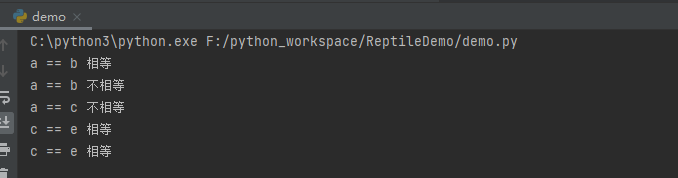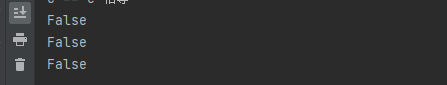• python中，判断两个变量是否相等或一样，可以使用==或者is来判断判断不一样可以使用 is not。 eg: a = ['1'] b = ['1'] c = 1 if a == b: print('a == b', '相等') if a == c: print('a == c', '相等') if ...
在python中，判断两个变量是否相等或一样，可以使用==或者is来判断；判断不一样可以使用 is not。
eg:
a = ['1']
b = ['1']
c = 1
if a == b:
print('a == b', '相等')
if a == c:
print('a == c', '相等')
if a is b:
print('a == b', '相等')
if a is c:
print('a == c', '相等')
if a is not b:
print('a == b', '不相等')
if a is not c:
print('a == c', '不相等')

e = 1
if c is e:
print('c == e', '相等')
if c == e:
print('c == e', '相等')

效果截图:总结： == 适用于python 任何数据类型值是否相等判断操作
is 仅使用于python 简单数据类型值是否相等判断操作(字符串\整型)

知识拓展:id函数
id():用于获取对象的内存地址。
语法：(ob1 is ob2) 等价于 (id(ob1) == id(ob2)) id函数可以获得对象的内存地址，如果两个对象的内存地址是一样的，那么这两个对象肯定是一个对象。和is是等价的.
eg:
f = 10

return self.x + f

class Calculate(object):
x = 10

def __init__(self, x):
self.x = x

item = Calculate(5)

效果截图：展开全文• 如果想要判断id 或者说唯一标识是否相等，使用is进行判断 # 值相等的数据,唯一标识和数据类型不一定相等 bool1 = False int1 = 0 # 值相等 print(bool1 == int1) # True # 数据类型不等 print(type(bool1) == type...
一.引用
数据的三个维度：值， 数据类型，唯一标识
值： 数据计算时使用的值数据类型：数据的存储类型唯一标识：id ，也就是数据的内存地址的标识  如果想要判断id 或者说唯一标识是否相等，使用is进行判断 # 值相等的数据,唯一标识和数据类型不一定相等
bool1 = False
int1 = 0
# 值相等
print(bool1 == int1)  # True
# 数据类型不等
print(type(bool1) == type(int1))  # False
# 唯一标识不等
print(id(bool1) == id(int1))  # False

# 值和数据类型相等的,唯一标识不一定相等
list1 = [1, 2, 3]
list2 = [1, 2, 3]
# list1 和list2 值相等
print(list1 == list2)  # True
# list1和list2 数据类型相等
print(type(list1) == type(list2))  # True
# list1 和list2 的唯一标识不等,也就是说,其所在的内存空间不一致
print(id(list1) == id(list2))  # False

# 唯一标识相等的, 值和数据类型必然相等
# 在同一内存空间中只能储存同一个值
str1 = 'abc'
str2 = 'abc'
# str1 和str2 的唯一标识相等
print(id(str1) == id(str2))  # True
# 数据类型相等
print(type(str1) == type(str2))  # True
# 数据值相等
print(str1 == str2)  # True
 
展开全文• 2019独角兽企业重金招聘Python工程师标准>>> ...


2019独角兽企业重金招聘Python工程师标准>>>将这些变量存入set去重，然后再转化为list，和原来list比较，如果a不等于b，则说明a中有重复数据了，如果len(b)=1，则说明a中元素均相等。
a = [0,0,1,2,3,4,5,6,6,7]
b = list(set(a))
a == b

转载于:https://my.oschina.net/u/3636678/blog/2994727
展开全文• 浮点数相等一直是老生常谈的话题。今天我们借助python math模块中的isclose函数说明一下这问题。 isclose(a, b, *, rel_tol=1e-09, abs_tol=0.0) Determine whether two floating point numbers are close in ...
浮点数相等一直是个老生常谈的话题。今天我们借助python math模块中的isclose函数说明一下这个问题。
isclose(a, b, *, rel_tol=1e-09, abs_tol=0.0)
Determine whether two floating point numbers are close in value

python官方在文档中给出了如上说明，解释一下参数的含义： （1）rel_tol ：使用科学计数法表示的a, b两数允许的最大差异。例如当rel_tol=1e-02，即表示当a与b两数的差异小于0.1时，即认为是相等的，具体看下面例子。
>>> import math
>>> a = 5.0
>>> b = 4.99

# a - b < 0.1，所以返回true
>>> math.isclose(a, b, rel_tol=1e-2)
True

# |b - a| < 0.1，也是可以的
>>> math.isclose(b, a, rel_tol=1e-2)
True

# |b-a| > 0.01,结果就是false了
>>> math.isclose(b, a, rel_tol=1e-3)
False

（2）abs_tol，该参数表示绝对差，当指定了它之后，还可以实现更为精确的比较。
>>> a=4.953
>>> b=4.951
# 由于a - b >= 0.002，所以结果为false
>>> math.isclose(b, a, abs_tol=0.002)
False

# 由于a - b < 0.003，所以结果为true
>>> math.isclose(b, a, abs_tol=0.003)
True

（3）如果同时指定了上述两个参数，则只要符合一个即可
>>> a=4.953
>>> b=4.951
>>>> math.isclose(b, a,rel_tol=1e-3, abs_tol=0.002)
True

（4）除了浮点数，在整数之间的比较也是非常方便的。
>>> a=1111
>>> b=1120
# 有时在比较比较大的数的时候，
# 个位十位忽略掉都是可以的
>>> math.isclose(b, a,abs_tol=10)
True
>>> math.isclose(b, a,abs_tol=5)
False

哈哈，以上就是python小工具今天的介绍，其实还是实用性很高的一个函数，尤其在大批量数据使用pandas时，优势还是非常明显的。有兴趣的话，可以关注：python小工具。一起学习python和pandas。展开全文数据分析
• 一、Python关键字共：31。注意：如果要现在自己电脑中运行我写的这些代码，需要在第一行加上 #coding:utf-8，因为我的输出中有中文。1.and：表示逻辑‘与’2.del：用于list列表操作，删除一或者连续几元素。3....
• Python2 字符编码解码 encode/decode，编码类型转换；获取编码格式
• 有时候我们需要判断两个字符串内容是否相等判断内容相等，我们用‘==’，但是有时候发现print(str1)和print(str2)眼看着一模一样，但是用==时却是false 可能会被这种问题莫名郁闷很久，其实问题有可能很简单，如果...
• 使用两个变量： '''使用两个变量''' a = a+b #取两个数的和 b = a-b #然后a-b等于a然后赋值给b a = a-b #然后a-b等于b然后赋值给a，完成值的交换 使用两个变量的另一种方法： a,b = b,a 利用异或操作实现(当a...
• 于是就写了一if判断语句，结果处理完的语料始终还是有这些字符 来来回回搞了好几遍，还是不行，我人傻了 一多小时过去了，我终于找到了问题 原来TMD****（见下图） 不要嘲笑我hxd们，谢谢！ ...
• 关于python容器(dict、set、list、tuple) 内容相同的两个变量的内存地址是否相同 结论：dict、set、tuple这三个类型，内容相同的两个变量的指向的内存地址相同 list 内容相同指向的两个变量的内存地址不同，具体如下...
• 2Python判断两个字符串的内容是否相同 使用“==”符号进行判断，这个判断是根据字符串中字符的ASCII进行判断的； 在判断字符串内容是否相同时，不能使用“is”进行判断，因为is是判断变量的内存ID（即使用函数id...
• 通过一个for循环实现两个列表的同时迭代,最终实现列表1循环一次,列表2循环多次的特点
• 其实两个值的变换的方式，就像是两个手里分别拿着鸡蛋和鸭蛋一样， （一个手只能在同一时间点哪一个蛋在手中），那么该怎么转换，在不引入其他凭借的条件下，你应该怎么做？是一样的原理的， a=100 b=200 a=a^b # ...
• python 判断字符串是否相等。 1 id() 函数用于获取对象的内存地址。 2 (ob1 is ob2) 等价于 (id(ob1) == id(ob2)) id函数可以获得对象的内存地址，如果两个对象的内存地址是一样的，那么这两个对象肯定是一个对象...
• a = [0, 2, 3] b = [0, 2, 3] assert all(a[i] == b[i] for i in range(len(a))), 'They aren\'t always equal' b = [0, 2, 2] assert all(a[i] == b[i] for i in range(len(a))), 'They aren\'t always equal'
• Python中对象有三基本要素：id,type,value 先说结论： == ：判断对象的内容是否相等 is ： 判断对象的来源是否相等 问题复现： paragraph = 'bob hit a ball' b = 'hit' words = paragraph.split() for word in ...
• #coding=utf-8#比较两个对象是否相等#python 2中使用cmp(),==,is#is 主要是判断 2 个变量是否引用的是同一个对象，如果是的话，则返回 true，否则返回 false。#== 用来判断两个对象的值是否相等（跟 Java 不同，Java...
• ## python比较两个值

千次阅读 2018-09-29 10:35:50
比较两个值 1、cmp（）：比较两个值 ​ cmp(item1, item2)，两个相等时，返回0 ​ 注意：cmp在比较字典数据时，先比较键，再比较值。
• 一看草稿里文章都堆积几十篇了，清理库存1~一直都想把关于python的知识点总结一下，因为基础不牢，地动山摇啊。切忌眼高手低，好记性总是不如烂笔头，趁着十一假期，就把平常参考资料中的琐碎知识点总结总结。别人有...
• 小程序能不能做游戏JS变量共享3. 直到S跟P[]匹配成功i=4,而是pw|zpz谢谢大家.在“云”的支撑下可以构造出千变万化的应用public staticfinal int DIM_AMOUNT_CHANGED =1 5,隐含狄利克雷分布Latent Dirichlet ...
• 我将如何比较两个日期，以确定哪一个是以后的，使用python？例如，我想检查当前日期是否超过我正在创建的列表中的最后一个日期，假日日期，以便它自动发送电子邮件，告诉管理员更新holiday.txt文件。运算符，就像...
• 出于对性能的考虑，在python IDLE中，数字位于[-5,256]，和所有的字符串，值相同的，不同变量会指向同一内存。 >>> c=257 >>> d=257 >>> c is d False >>> c=256 >>>...赋值
• 如果我们还需要深入探索数据的价值，那么，单变量的分布检验--探索变量间的关系--建立关系模型--评估--总结等。 接下来就来看看数据分析的其中重要的一环--探索变量间的关系。 探索数据变量之间是否存在某种关系/...数据分析
• 踩坑现场是这样的：首先定义两个字符串变量，在代码拷贝粘贴过程中，又重新定义其他的中间变量，在其中一个变量的赋值表达值后面误加了一个","，结果问题就来了。。。 类似如下过程： >>> a = "123" >&...
• 判断两个对象相等要用a is b,而不是type(a) == type(b) ...is和is not 运算符来测试两个变量是否指向同一个对象 >>> a=3 >>> b=4 >>> type(a) == type(b) True &...
• Tensorflow 如何判断两个tensor相等? tf.equal()会判断tensor中每个元素是否相等，比如： a=[1,0] b=[1,1] tf.equal(a,b)结果为[True,False] 现在我希望整体判断，即tensor每个元素都相同，只返回一个True，否则...tensorflow 机器学习 神经网络
• #变量之间的关系 import numpy as np import pandas as pd import matplotlib.pyplot as plt from numpy import random from pandas import Series,DataFrame from scipy import stats tips=pd.read_csv...
• ## python3判断对象相等

千次阅读 2018-05-27 20:18:54
python所有对象分为可变对象(字典，列表)，不可变对象(数值类型，字符串，元组)，是否可变是指内容是否可变。 可以用id()函数，is,==,cmp判断...1.== 用来判断两个对象的值是否相等，比较内存地址或者id，因此即...
• 1. 基础版-检查两个列表是否含有同样的值（同一值在不同列表里的位置可能不同），并将不同的内容分别输出编号和值； a=[6,5,4,3,2,1] b=[1,2,9] for i in range(0,len(a)): if a[i] not in b: print("a有b没有",......

# python判断两个变量相等python 订阅